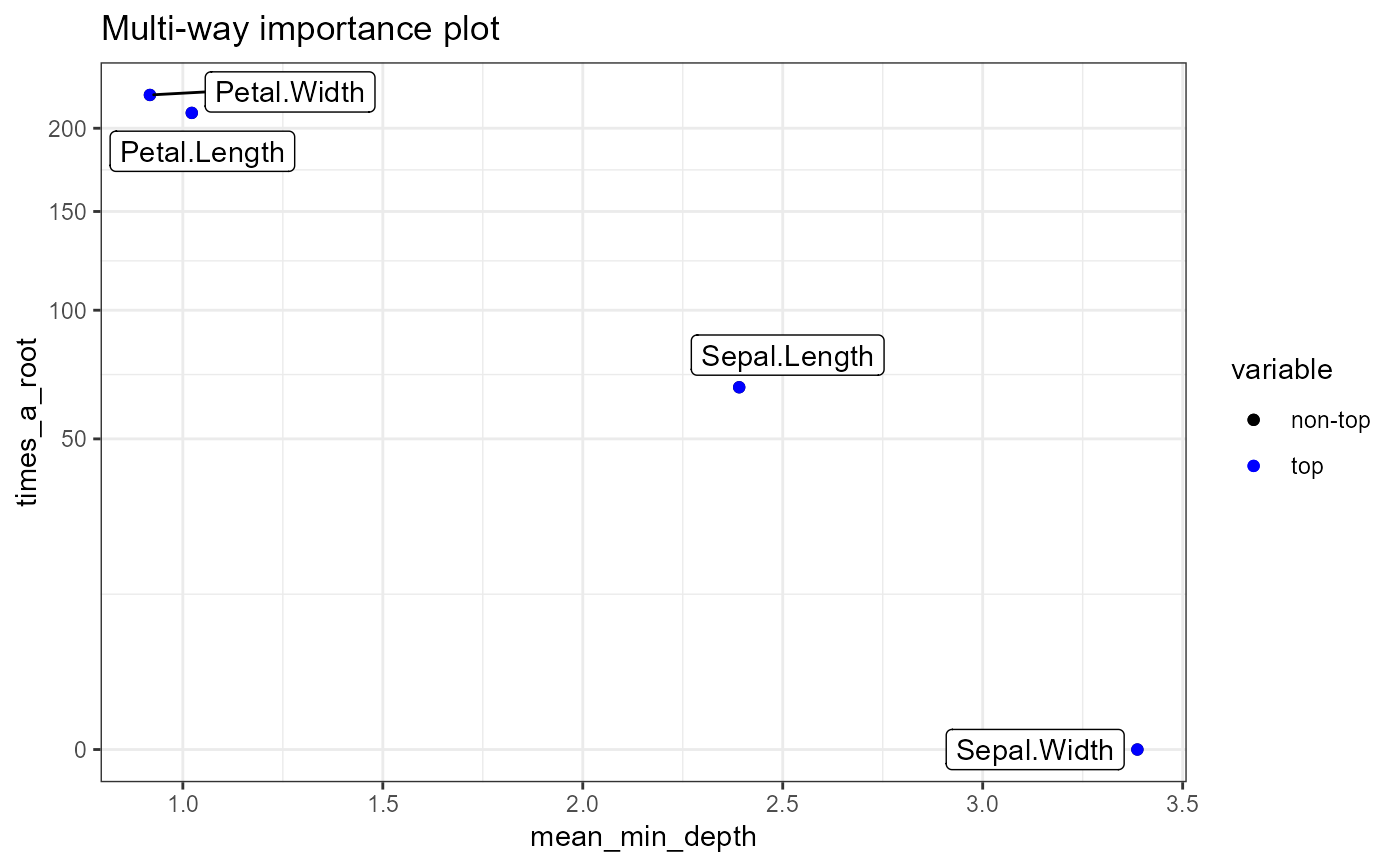Plot two or three measures of importance of variables in a random fores. Choose importance measures from the colnames(importance_frame).

plot_multi_way_importance(
importance_frame,
x_measure = "mean_min_depth",
y_measure = "times_a_root",
size_measure = NULL,
min_no_of_trees = 0,
no_of_labels = 10,
main = "Multi-way importance plot"
)

## Arguments

importance_frame A result of using the function measure_importance() to a random forest or a randomForest object The measure of importance to be shown on the X axis The measure of importance to be shown on the Y axis The measure of importance to be shown as size of points (optional) The minimal number of trees in which a variable has to be used for splitting to be used for plotting The approximate number of best variables (according to all measures plotted) to be labeled (more will be labeled in case of ties) A string to be used as title of the plot

A ggplot object

## Examples

forest <- randomForest::randomForest(Species ~ ., data = iris, localImp = TRUE)
plot_multi_way_importance(measure_importance(forest))